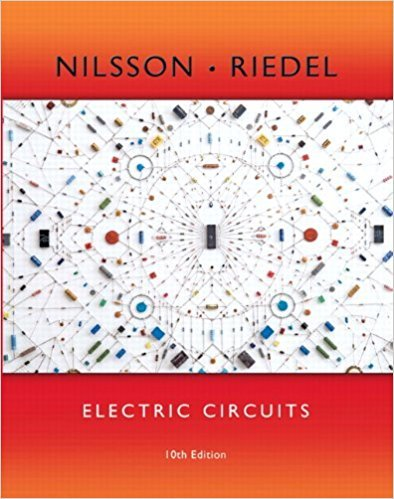×
×

# Solutions for Chapter 10.3: The rms Value and Power Calculations## Full solutions for Electric Circuits | 10th Edition

ISBN: 9780133760033Solutions for Chapter 10.3: The rms Value and Power Calculations

Solutions for Chapter 10.3
4 5 0 337 Reviews
13
2
##### ISBN: 9780133760033

This textbook survival guide was created for the textbook: Electric Circuits, edition: 10. This expansive textbook survival guide covers the following chapters and their solutions. Electric Circuits was written by and is associated to the ISBN: 9780133760033. Since 1 problems in chapter 10.3: The rms Value and Power Calculations have been answered, more than 13379 students have viewed full step-by-step solutions from this chapter. Chapter 10.3: The rms Value and Power Calculations includes 1 full step-by-step solutions.

Key Engineering and Tech Terms and definitions covered in this textbook
×